# Constructive Geometry for Complex Grobs

Version 1: Tuesday 31 May 2022This document describes developments in the `grobCoords()` function in the R package 'grid', plus improvements to the 'gridGeometry' package that makes use of the `grobCoords()` function.

These features are available in R version 4.2.0 and in 'gridGeometry' version 0.3-0.

## 1. Introduction

The `grobCoords()` function (introduced in R version 3.6.0) generates a set of coordinates from a 'grid' grob. For example, the following code defines a 'grid' rectangle grob (and draws it) then calculates a set of coordinates from this rectangle grob (the vertices of the rectangle). The rectangle is centred 1 inch in from the left of the image and 1 inch up form the bottom of the image and is 1 inch square, so the vertices are at (0.5, 0.5), (0.5, 1.5), (1.5, 1.5), and (1.5, 0.5).

```library(grid)
```
```rectangle <- rectGrob(1, 1, 1, 1, default.units = "in", name = "r")
grid.draw(rectangle)
```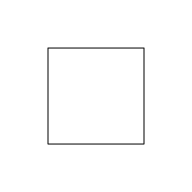```coords <- grobCoords(rectangle, closed = TRUE)
coords
```
```  grob r
shape 1
x: 0.5 0.5 1.5 ... [4 values]
y: 0.5 1.5 1.5 ... [4 values]
```

The 'gridGeometry' package (Murrell, 2022a) combines 'grid' grobs using operators like "union" and "intersection". For example, in the following code we define a triangle shape (and draw it) then we call `grid.polyclip()` from 'gridGeometry' to draw the union of the rectangle from above with this triangle.

```library(gridGeometry)
```
```triangle <- polygonGrob(c(1, 2, 2), c(1, 1.5, .5), default.units="in")
grid.draw(triangle)
```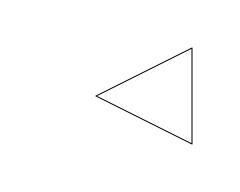```grid.polyclip(rectangle, triangle, "union")
```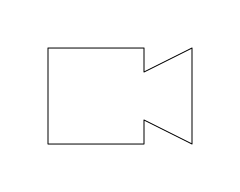The 'gridGeometry' package works by getting coordinates for each grob, via `grobCoords()`, and combining those coordinates using the 'polyclip' package (Johnson and Baddeley, 2019). Any changes to the `grobCoords()` function require changes to the 'gridGeometry' package.

The examples above are straightforward because, in the first case, we calculated coordinates from a grob that draws a single shape, so the result was a single set of (x, y) coordinates. In the second case, we combined two grobs that both draw a single shape, so 'gridGeometry' only had to combine one shape with another.

This report explores scenarios that are more complex, where we want to use `grobCoords()` to obtain the coordinates for a grob that draws more than one shape and where we want to use 'gridGeometry' to combine grobs that each draw more than one shape.

## 2. Improving `grobCoords()`

Before we consider more complex scenarios, we need to take a closer look at the coordinates generated for a grob that draws a single shape (shown again below).

```coords
```
```  grob r
shape 1
x: 0.5 0.5 1.5 ... [4 values]
y: 0.5 1.5 1.5 ... [4 values]
```

We have a single set of (x, y) coordinates (four points representing the vertices of the rectangle), but we can also see that those coordinates belong to "shape 1" and that shape belongs to "grob r". The "r" comes from the `name` argument that we supplied in the original call to `rectGrob()`.

This simple example demonstrates one of the changes to `grobCoords()` in R 4.2.0: the return value used to be just a list of lists with components `x` and `y` (a list of "xy-lists"), but now the return value is a "GridGrobCoords" object, with additional information (such as names), and a print method.

```class(coords)
```
```   "GridGrobCoords"
```

That additional information becomes more important when we work with a grob that draws more than one shape. For example, the following code defines a grob that describes two rectangles (and draws them) and then calculates the coordinates from that grob. The result shows coordinates for two shapes, both of which belong to "grob r2".

```rectangles <- rectGrob(c(1, 2.5), 1, 1, 1, default.units = "in", name = "r2")
grid.draw(rectangles)
```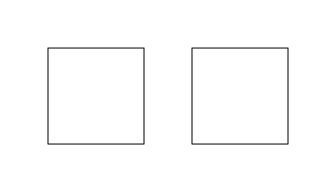```grobCoords(rectangles, closed = TRUE)
```
```  grob r2
shape 1
x: 0.5 0.5 1.5 ... [4 values]
y: 0.5 1.5 1.5 ... [4 values]
shape 2
x: 2 2 3 ... [4 values]
y: 0.5 1.5 1.5 ... [4 values]
```

The following code demonstrates a different scenario. Here we define a path grob that consists of two rectangles, but the two rectangles together define a single shape; the inner rectangle creates a hole in the outer rectangle. There are two sets of (x, y) coordinates again, but this time they both belong to "shape 1". We can also see that the fill rule ("evenodd") has been recorded in the "GridGrobCoords" object.

```x <- c(.5, .5, 1.5, 1.5, .75, .75, 1.25, 1.25)
y <- c(.5, 1.5, 1.5, .5, .75, 1.25, 1.25, .75)
path <- pathGrob(x, y,
id = rep(1:2, each=4), rule = "evenodd",
default.units = "in", name = "p",
gp = gpar(fill = "grey"))
grid.draw(path)
```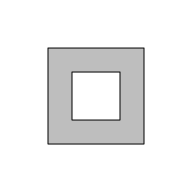```grobCoords(path, closed = TRUE)
```
```  grob p  (fill: evenodd)
shape 1
x: 0.5 0.5 1.5 ... [4 values]
y: 0.5 1.5 1.5 ... [4 values]
shape 1
x: 0.75 0.75 1.25 ... [4 values]
y: 0.75 1.25 1.25 ... [4 values]
```

Of course, it is also possible to have a grob that describes multiple paths, each of which consists of multiple sets of coordinates, as shown below. In this case we have a path grob that describes two shapes, each of which consists of two rectangles, with an inner rectangle that creates a hole in an outer rectangle. There are four sets of coordinates corresponding to the four rectangles, but two sets of coordinates belong to shape 1 and two sets of coordinates belong to shape 2.

```paths <- pathGrob(c(x, x + 1.5), c(y, y),
id = rep(rep(1:2, each=4), 2),
pathId = rep(1:2, each=8), rule = "evenodd",
default.units = "in", name = "p2",
gp = gpar(fill = "grey"))
grid.draw(paths)
```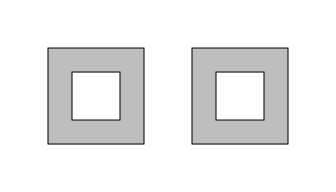```grobCoords(paths, closed = TRUE)
```
```  grob p2  (fill: evenodd)
shape 1
x: 0.5 0.5 1.5 ... [4 values]
y: 0.5 1.5 1.5 ... [4 values]
shape 1
x: 0.75 0.75 1.25 ... [4 values]
y: 0.75 1.25 1.25 ... [4 values]
shape 2
x: 2 2 3 ... [4 values]
y: 0.5 1.5 1.5 ... [4 values]
shape 2
x: 2.25 2.25 2.75 ... [4 values]
y: 0.75 1.25 1.25 ... [4 values]
```

We can add another level of complexity by considering 'grid' gTrees, which are collections of grobs. For example, the following code defines a gTree consisting of a rectangle and a path, draws the gTree, and calculates its coordinates. The result has an extra level: one set of coordinates belongs to a "shape 1" that belongs to "grob r", two other sets of coordinates belong to a "shape 1" that belongs to "grob p3", and both "grob r" and "grob p3" belong to "gTree parent".

```path2 <- pathGrob(x + 1.5, y,
id = rep(1:2, each=4), rule = "evenodd",
default.units = "in", name = "p3",
gp = gpar(fill = "grey"))
gt <- gTree(children=gList(rectangle, path2), name = "parent")
grid.draw(gt)
```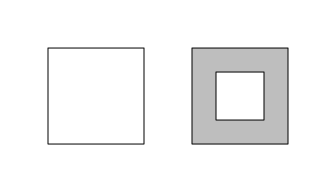```grobCoords(gt, closed = TRUE)
```
```  gTree parent
grob r
shape 1
x: 0.5 0.5 1.5 ... [4 values]
y: 0.5 1.5 1.5 ... [4 values]
grob p3  (fill: evenodd)
shape 1
x: 2 2 3 ... [4 values]
y: 0.5 1.5 1.5 ... [4 values]
shape 1
x: 2.25 2.25 2.75 ... [4 values]
y: 0.75 1.25 1.25 ... [4 values]
```

We could keep going and add further layers, because the children of a gTree can themselves be gTrees, but hopefully it is now clear how that would go. We will turn instead to an example of how the coordinates that `grobCoords()` returns can be used, by looking at the latest changes to the 'gridGeometry' package.

## 3. Improving 'gridGeometry'

As we saw at the start, the 'gridGeometry' package can be used to combine grobs. The following code shows another simple example where we create a new shape by subtracting a circle from a rectangle. We first draw the two grobs separately to show what they look like and then we draw the result of the rectangle "minus" the circle. We fill the result with grey to show that the circle has punched a hole in the rectangle.

```circle <- circleGrob(1, 1, r = .3,
default.units = "in", gp=gpar(fill = NA))
grid.draw(rectangle)
grid.draw(circle)
```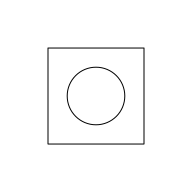```grid.polyclip(rectangle, circle, "minus",
gp=gpar(fill = "grey"))
```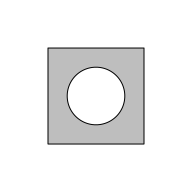The following code demonstrates a more complex scenario with a circle grob that draws three circles. We first draw the circles on top of the rectangle to show the shapes that we are dealing with.

```circle <- circleGrob(c(.5, 1, 1.5), 1, r = .3,
default.units = "in", gp=gpar(fill = NA))
grid.draw(rectangle)
grid.draw(circle)
```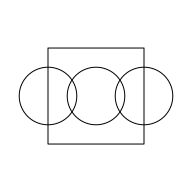When we subtract this circle grob from the rectangle grob, the result is obtained by first "reducing" the three circles to a single shape and then subtracting that result from the rectangle. The result in this case is the rectangle with the three circles removed from it. More accurately, the result is the rectangle with the union of the three circles subtracted from it.

```grid.polyclip(rectangle, circle, "minus",
gp=gpar(fill = "grey"))
```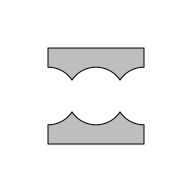When we give `grid.polyclip()` a grob that draws multiple shapes, the grob is first reduced to a single shape before being combined with the other argument. By default, this reduction occurs by using `polyclip()` to combine the shapes using a "union" operator. In the example above, the three circles are reduced via "union" to the single shape below.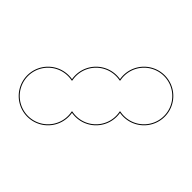There are two new arguments to `grid.polyclip()`, `reduceA` and `reduceB`, which can be used to control how multiple shapes are reduced to a single shape. For example, the following code reduces the three circles using "xor" before subtracting them from the rectangle.

```grid.polyclip(rectangle, circle, "minus", reduceB = "xor",
gp=gpar(fill = "grey"))
```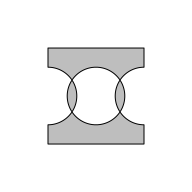There is also a new function, `grid.reduce()`, that just performs the grob reduction. This function takes a grob and reduces it to a new grob that describes a single shape (either a path or a line). For example, the following code reduces the grob that draws three circles into a single shape using "xor". The grey filled area below is the shape that was subtracted from the rectangle to produce the `grid.polyclip()` result above.

```grid.reduce(circle, "xor", gp=gpar(fill="grey"))
```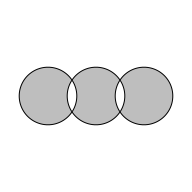In the more complex example above, only the second argument to `grid.polyclip()` was a grob that draws more than one shape. It is also possible for the first argument to `grid.polyclip()` to be a grob that draws more than one shape and it is possible for either or both arguments to be gTrees. In the case of gTrees, each child grob is reduced and then all of the reduced children are reduced together.

The following example provides a demonstration of collapsing a more complex grob. For this example, we will work with the SVG version of the R logo (shown below).The following code uses the 'grImport2' package (Potter and Murrell, 2019) to import the R logo into a 'grid' gTree. We create a gTree that will just draw the outlines of the imported logo and fill it with a semitrasparent grey. The 'rsvg' package (Ooms, 2022) is used to convert the original SVG logo into a Cairo graphics version in preparation for import.

```library(rsvg)
rsvg_svg("Rlogo.svg", "Rlogo-cairo.svg")
library(grImport2)
semigrey <- rgb(.5, .5, .5, .5)
logoGrob <- pictureGrob(Rlogo,
gpFUN = function(gp) gpar(col="black", fill=semigrey))
```

The resulting gTree contains another gTree, which contains two further gTrees ("picComplexPath" gTrees), each of which contains two grobs (a "picPath" grob and a "picPolyline" grob).

```grid.ls(logoGrob)
```
```  import.1.GRID.gTree.35
GRID.gTree.34
GRID.picComplexPath.28
GRID.picPath.29
GRID.picPolyline.30
GRID.picComplexPath.31
GRID.picPath.32
GRID.picPolyline.33
```

Drawing the logo shows that there are two main shapes in the logo, the "R" itself and the ellipse that encircles the top of the "R" and that both of those shapes consist of two curves with the inner curve creating a hole in the outer curve.

```grid.draw(logoGrob)
```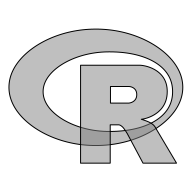The following code calls `grid.reduce()` to convert that complicated gTree to a single shape (using the default "union" operator). This forms the union of the "R" shape with the ellipse shape.

```grid.reduce(logoGrob, gp=gpar(fill="grey"))
```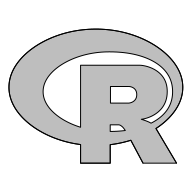The following code "xor"s the R logo gTree with the rectangle that we used in previous examples. This implicitly performs the reduction of the R logo (that we just did explicitly) before subtracting it from the rectangle.

```grid.polyclip(rectangle, logoGrob, "xor", gp=gpar(fill="grey"))
```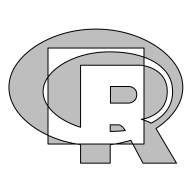### Combining "open" shapes

All of the examples so far have involved "closed" shapes - shapes that have an interior that can be filled, like polygons and paths. It is also possible for the `A` argument to `grid.polyclip()` to be an "open" shape, like a line segment or a Bezier curve.

The following code demonstrates a simple example where we subtract a single circle from a single line segment. As before, we first draw both shapes separately and then we draw the result of `grid.polyclip()`.

```line <- segmentsGrob()
circle <- circleGrob(1, 1, r = .3,
default.units = "in", gp=gpar(fill = NA))
grid.draw(circle)
grid.draw(line)
```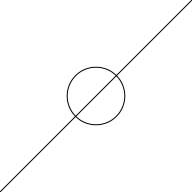```grid.polyclip(line, circle, "minus")
```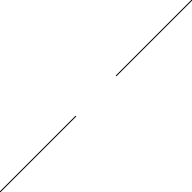The next example shows what happens when we have multiple shapes from an open grob. In this case we have a segments grob that draws two lines that criss-cross each other and we are subtracting a single circle.

```lines <- segmentsGrob(0:1, 0, 1:0, 1)
circle <- circleGrob(1, 1, r = .3,
default.units = "in", gp=gpar(fill = NA))
grid.draw(circle)
grid.draw(lines)
```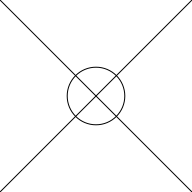```grid.polyclip(lines, circle, "minus")
```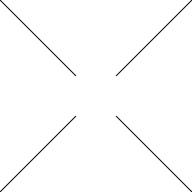Although that result may be what we expected, it hides a detail about how the `A` argument is reduced. This result does not come from reducing the two line segments with a "union" operator (the default that we saw happening for the `B` argument in previous examples). If we try to take the union of two open shapes, the result is empty, as shown below.

```grid.reduce(lines, "union")
```When the `A` argument is open, by default, it is reduced using the "flatten" operator rather than the "union" operator, which is the default for closed shapes. The "flatten" operator just combines all of the open shapes into a single grob.

```grid.reduce(lines)
```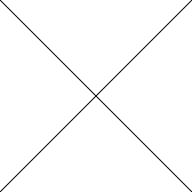The "flatten" operator can also be used for the `B` argument and for open shapes. The following code provides a simple demonstration. The segments grob is the same as in the last example, but we subtract a circle grob that draws two circles. We specify `reduceB = "flatten"` so that the two circles are reduced to a list of two sets of coordinates (one for each circle) and we specify `fillB = "evenodd"` so that the flattened circles are interpreted using an even-odd fill rule (so the inner circle creates a hole in the outer circle). The result is that we subtract a donut from the two line segments.

```lines <- segmentsGrob(0:1, 0, 1:0, 1)
circle <- circleGrob(1, 1, r = c(.3, .1),
default.units = "in", gp=gpar(fill = NA))
grid.draw(circle)
grid.draw(lines)
```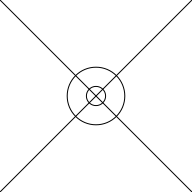```grid.polyclip(lines, circle, "minus", reduceB = "flatten", fillB = "evenodd")
```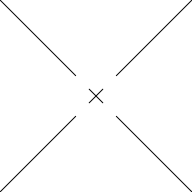### The `trim()` function

The 'gridGeometry' package also has a `trim()` function for extracting subsets of open shapes. For example, the following code extracts a subset of a line segment starting from .2 of the distance along the segment at ending at halfway along the line segment. The original line is drawn in grey and the subset is drawn in (thick) black.

```line <- segmentsGrob(.2 ,.2, .8, .8, gp=gpar(lwd=2, col="grey"))
grid.draw(line)
grid.trim(line, .2, .5, gp=gpar(lwd=5))
```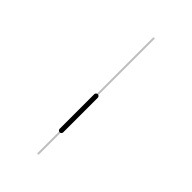The `grid.trim()` function has also been updated to handle grobs that draw more than one shape: all shapes are trimmed using the same set of `from` and `to` arguments. For example, the following code trims a segments grob that draws two line segments, using the same `from` and `to` as in the previous example.

```lines <- segmentsGrob(c(.2, .4), .2, c(.6, .8), .8,
gp=gpar(lwd=2, col="grey"))
grid.draw(lines)
grid.trim(lines, .2, .5, gp=gpar(lwd=5))
```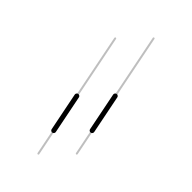The following code shows a more complex example where we trim a gTree that has the segments grob as its child, plus a circle grob, plus another gTree that has two lines grobs as its children. Again, all children are trimmed using the same set of `from` and `to` arguments. This also shows that closed shapes, like the circle, produce no output when trimmed. Only the open shapes are include in the result.

```gt <- gTree(children=gList(lines,
circleGrob(),
gTree(children=gList(linesGrob(c(.2, .2, .4),
c(.6, .8, .8)),
linesGrob(c(.6, .8, .8),
c(.2, .2, .4))))),
gp=gpar(lwd=2, col="grey", fill=NA))
grid.draw(gt)
grid.trim(gt, .2, .5, gp=gpar(lwd=5))
```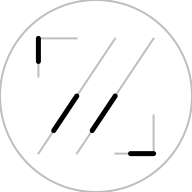## 4. More information about the changes to `grobCoords()`

The main change to the 'grid' functions `grobCoords()` and `grobPoints()` is that they now return more complex values. This section describes the format of those data structures for anyone who wants to write code that either generates or consumes these new values.

There are three new classes of object:

• "GridCoords" is a list with numeric components `x` and `y`.

This represents a set of coordinates that describe a simple shape or part of a more complex shape.

A "GridCoords" object can be generated with the `gridCoords()` function, which takes `x` and `y` as arguments.

```gc <- gridCoords(x=1:4, y=4:1)
gc
```
```  x: 1 2 3 ... [4 values]
y: 4 3 2 ... [4 values]
```
• "GridGrobCoords" is a list of one or more "GridCoords".

This represents the shapes that are described by a 'grid' grob.

The list can have names to indicate which "GridCoords" belong to the same shape (e.g., a single path consisting of two concentric circles). The list also has a `"name"` attribute and may have a `rule` attribute.

A "GridGrobCoords" object can be generated with the `gridGrobCoords()` function, which takes a list of "GridCoords" and a `name` argument and an optional `rule` argument.

```ggc <- gridGrobCoords(list("1"=gc), name="A")
ggc
```
```  grob A
shape 1
x: 1 2 3 ... [4 values]
y: 4 3 2 ... [4 values]
```
• "GridGTreeCoords" is a list of one or more "GridGrobCoords" or "GridGTreeCoords".

This represents the shapes that are described by a 'grid' gTree. The list has a `"name"` attribute.

A "GridGTreeCoords" object can be generated with the `GridGTreeCoords()` function, which takes a list of "GridGrobCoords" or "GridGTreeCoords" objects and a `name` argument.

```ggtc <- gridGTreeCoords(list(ggc), name="B")
ggtc
```
```  gTree B
grob A
shape 1
x: 1 2 3 ... [4 values]
y: 4 3 2 ... [4 values]
```
```ggtc2 <- gridGTreeCoords(list(ggtc, ggc), name="C")
ggtc2
```
```  gTree C
gTree B
grob A
shape 1
x: 1 2 3 ... [4 values]
y: 4 3 2 ... [4 values]
grob A
shape 1
x: 1 2 3 ... [4 values]
y: 4 3 2 ... [4 values]
```

The reason for introducing these more complex structures is that more information about the original grob or gTree is retained in the `grobCoords()` result. This makes it possible to identify which coordinates correspond to which shape within the grob or gTree. The Discussion mentions one example where this used within 'grid' itself (to resolve pattern fills).

The 'gridGeometry' package provides an interface between the 'grid' package and the 'polyclip' package. This requires converting from a 'grid' grob to a list of xy-lists that the 'polyclip' package can work with (and back again).

In R versions prior to 4.2.0, the `grobCoords()` function generated a list of xy-lists as its output, so the result from `grobCoords()` could be fed directly to functions in the 'polyclip' package. From R 4.2.0, the result from `grobCoords()` is more complex, so 'gridGeometry' has some additional functions to to convert `grobCoords()` output to a list of xy-lists.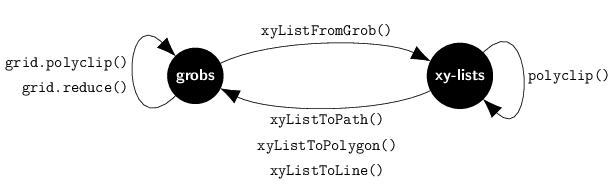The `grid.polyclip()` function and the `grid.reduce()` function accept 'grid' grobs and return 'grid' grobs. This is the simplest user interface.

The `xyListFromGrob()` function converts a grob into a list of xy-lists. It first converts a grob to a "GridGrobCoords" (or "GridGTreeCoords") object using `grobCoords()`. The "GridGrobCoords" (or "GridGTreeCoords") object is then reduced to a list of xy-lists either by "flatten"ing all of the "GridCoords" from the grob to a single list or by combining shapes (one or more "GridCoords" from the same grob) using `polyclip::polyclip()` and the operator specified in `reduceA` or `reduceB`. A gTree reduces each of its children and then combines the reduced children together. This function provides a to enter the 'polyclip' world of lists of xy-lists, starting from a 'grid' grob.

The functions `xyListToPath()`, `xyListtoPolygon()`, and `xyListToLine()` convert back from a list of xy-lists to a grob. There may be more than one xy-list, in which case, `xyListToPolygon()` creates a grob that draws a separate polygon for each xy-list, `xyListToPath()` creates a grob that draws a single path (using `rule` to determine the interior of the path), and `xyListToLine()` creates a grob that draws a separate line for each xy-list. These functions allow the user to return from the 'polyclip' world back to the world of 'grid' grobs.

The `polyclip()` function takes a list of xy-lists and returns a list of xy-lists. This allows the user to perform calculations in the 'polyclip' world. For example, we can use `xyListFromGrob()` to generate coordinates from a closed 'grid' grob, but then work with them as if they are coordinates from an open shape.

### Fill rules

When a list of xy-lists is fed to `polyclip::polyclip()`, a fill rule is specified to determine the interior of the shape that is described by the list of xy-lists. When we convert a grob to a list of xy-lists, the fill rule may be included in the `grobCoords()` result (e.g., if we are converting a path grob), but it may not. The fill rule that gets sent to `polyclip::polyclip()` is determined as follows: if the user specifies `fillA` or `fillB` explicitly, that fill rule is used; otherwise, if the `grobCoords()` result contains a fill rule, that is used; otherwise the fill rule is "nonzero" (the 'polyclip' way of saying "winding"). Note that this is different from the default of "evenodd" that `polyclip::polyclip()` itself uses.

### Combinations of open and closed shapes

The `grobCoords()` function has a `closed` argument to indicate whether we want the coordinates of a closed shape or an open shape. From R 4.3.0 or from 'gridGeometry' 0.3-1, where it can be determined that the grob is open, `closed` defaults to `FALSE`. Otherwise, `closed` defaults to `TRUE`. Prior to that, the `closed` argument must be specified explicitly.

When we ask for the coordinates from a polygon grob, we get the coordinates of the polygon if `closed=TRUE`, but we get nothing ("empty" coordinates) if `closed=FALSE`. Similarly, if we ask for the coordinates from a line grob, we get the coordinates of the line if `closed=FALSE`, but we get nothing if `closed=TRUE`. A gTree presents a problem because it can contain grobs that draw both open and closed shapes.

The `grid.polyclip()` function handles this problem by generating open coordinates for `A` and combining them with `B` and then also generating closed coordinates for `A` and combining them with `B`. The final result is then a gTree that combines the open result and the closed result.

The following code shows an example where `A` is a gTree consisting of a line and a rectangle and `B` is a circle grob (and the operator is "minus"). The result is a combination of the (closed) rectangle minus the (closed) circle and the (open) line minus the (closed) circle.

```grid.polyclip(gTree(children=gList(rectGrob(width=.5, height=.5),
segmentsGrob(0, .5, 1, .5))),
circleGrob(r=.2),
"minus",
gp=gpar(fill="grey"))
```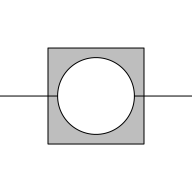Note that `B` cannot be open, a limitation imposed by the underlying Clipper library (Johnson, 2019). On the other hand, the Clipper library does allow `A` to be a combination of open and closed shapes (though the semantics of that can be tortuous), whereas 'gridGeometry' only ever calls `polyclip::polyclip()` with either `A` entirely closed or `A` entirely open.

### To reduce or not to reduce

By default, any grob (including gTrees) that draws more than one shape will be reduced. When `closed=TRUE`, the result will be a single shape based on the union of the multiple shapes. The 'polyclip' package (and the Clipper library) will accept a list of xy-lists, i.e., multiple shapes, so should we always reduce multiple shapes to a single shape? By default, we do always reduce, but the user has the option of specifying `op="flatten"`, which will result in sending multiple shapes to 'polyclip'.

## 6. Discussion

The main idea behind the changes to grobCoords is to provide more detailed and comprehensive information about the coordinates for a 'grid' grob. We want to retain information about where the sets of coordinates came from through both a hierarchical structure and labelling of components within that structure.

The 'gridGeometry' package makes some use of that extra information, e.g., to determine the fill rule that it sends to 'polyclip' functions.

The grobCoords() function is also used by 'grid' itself for resolving fill patterns (Murrell, 2022b), by making use of the names on `grobCoords()` output. In that case, the extra information is important because it allows us to resolve a pattern relative to individual shapes within a grob as well as relative to the bounding box around all shapes within a grob.

It is also hoped that the extra information provided by `grobCoords()` may prove useful to code writers and package developers that make use of `grobCoords()` output. One speculative application is for graphics device packages that do not natively support some of the new graphics engine features, like affine transformations, to add support for some features by working with grob coordinates. For example, a transformed circle could be produce by calculating the coordinates of the original circle, transforming the coordinates, and drawing the transformed coordinates as a polygon.

## 7. Technical requirements

The examples and discussion in this report relate to R version 4.2.0 and 'gridGeometry' version 0.3-1.

This report was generated within a Docker container (see Resources section below).

## 8. Resources

• The raw source file for this report, a valid XML transformation of the source file, a 'knitr' document generated from the XML file, two R files and the bibtex file that are used to generate the table of contents and reference sections, two XSL files and an R file that are used to transform the XML to the 'knitr' document, and a Makefile that contains code for the other transformations and coordinates everything. These materials are also available on github.
• This report was generated within a Docker container. The Docker command to build the report is included in the Makefile above. The Docker image for the container is available from Docker Hub; alternatively, the image can be rebuilt from its Dockerfile.

## How to cite this report

Murrell, P. (2022). "Constructive Geometry for Complex Grobs" Technical Report 2022-02, Department of Statistics, The University of Auckland. Version 1. [ bib | DOI | http ]

## 9. References

[Johnson, 2019]
Johnson, A. (2019). Clipper - an open source freeware library for clipping and offsetting lines and polygons. http://www.angusj.com/delphi/clipper.php. Accessed: 2019-02-27. [ bib ]
Johnson, A. and Baddeley, A. (2019). polyclip: Polygon Clipping. R package version 1.10-0. [ bib | http ]
[Murrell, 2022a]
Murrell, P. (2022a). gridGeometry: Polygon Geometry in 'grid'. https://github.com/pmur002/gridgeometry, https://stattech.wordpress.fos.auckland.ac.nz/2019/03/04/2019-01-a-geometry-engine-interface-for-grid/. [ bib ]
[Murrell, 2022b]
Murrell, P. (2022b). Vectorised pattern fills in r graphics. Technical Report 2022-01, Department of Statistics, The University of Auckland. version 1. [ bib | DOI | http ]
[Ooms, 2022]
Ooms, J. (2022). rsvg: Render SVG Images into PDF, PNG, (Encapsulated) PostScript, or Bitmap Arrays. R package version 2.3.1. [ bib | http ]
[Potter and Murrell, 2019]
Potter, S. and Murrell, P. (2019). grImport2: Importing 'SVG' Graphics. R package version 0.2-0. [ bib | http ]
[R Core Team, 2019]
R Core Team (2019). R: A Language and Environment for Statistical Computing. R Foundation for Statistical Computing, Vienna, Austria. [ bib | http ]